## Tamilnadu Samacheer Kalvi 6th Maths Solutions Term 3 Chapter 1 Fractions Ex 1.1

6th Maths Guide Term 3 Question 1.
Fill in the blanks.
(i) 7$$\frac{3}{4}$$ + 6$$\frac{1}{2}$$ = _______
(ii) The sum of whole number and a proper fraction is called ______
(iii) 5$$\frac{1}{3}$$ – 3$$\frac{1}{2}$$ = ______
(iv) 8 ÷ $$\frac{1}{2}$$ = ______
(v) The number which has its own reciprocal is _______.
Solution:
(i) 14$$\frac{1}{4}$$
(ii) Mixed Fraction
(iii) 1$$\frac{5}{6}$$
(iv) 16
(v) 1

Samacheer Kalvi 6th Maths Book Solutions Question 2.
Say True or False
(i) 3$$\frac{1}{2}$$ can be written as 3 + $$\frac{1}{2}$$.
(ii) The sum of any two proper fractions is always an improper fraction.
(iii) The mixed fraction of $$\frac{13}{4}$$ is 3$$\frac{1}{4}$$.
(iv) The reciprocal of an improper fraction is always a proper fraction.
(v) 3$$\frac{1}{4}$$ × 3$$\frac{1}{4}$$ = 9$$\frac{1}{16}$$
Solution:
(i) True
(ii) False
(iii) True
(iv) True
(v) False

6th Maths Guide 3rd Term Question 3.
Solution:
(i) Find the sum of $$\frac{1}{7}$$ and $$\frac{3}{9}$$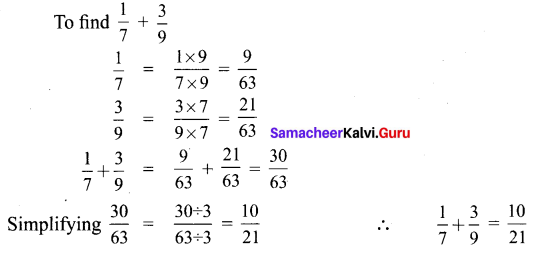(ii) What is the total of 3$$\frac{1}{3}$$ and 4$$\frac{1}{6}$$.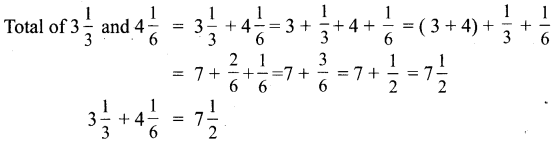(iii) Simplify : 1$$\frac{3}{5}$$ + 5$$\frac{4}{7}$$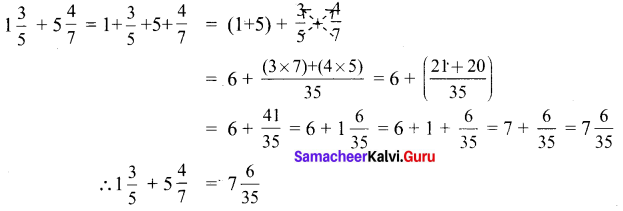(iv) Find the difference between $$\frac{8}{9}$$ and $$\frac{2}{7}$$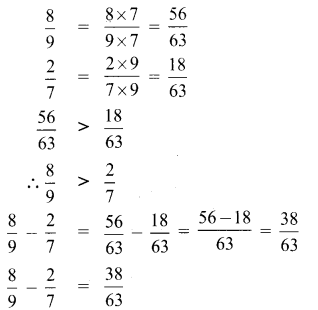(v) Subtract 1$$\frac{3}{5}$$ and 2$$\frac{1}{3}$$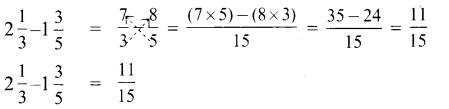(vi) Simplify: 7$$\frac{2}{7}$$ – 3$$\frac{4}{21}$$

Samacheer Kalvi 6th Maths Book Solutions Term 3 Question 4.
Convert mixed fraction into improper fractions and vice versa: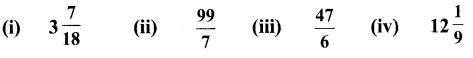Solution: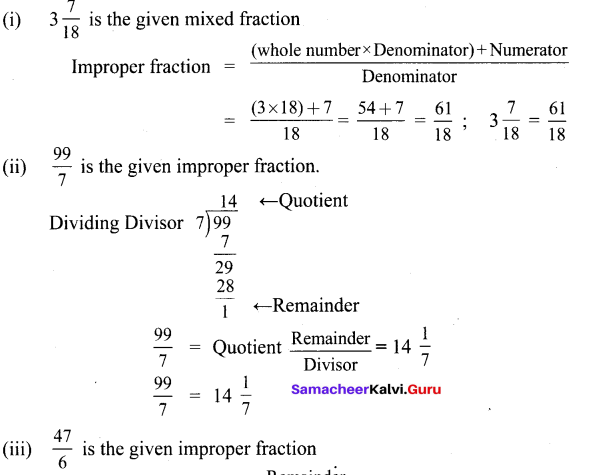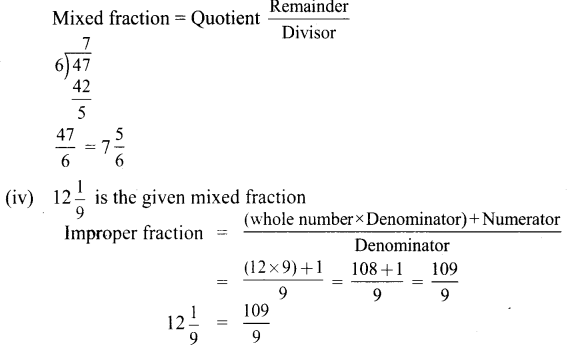6th 3rd Term Maths Guide Question 5.
Multiply the following :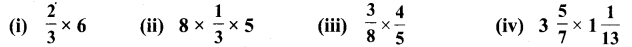Solution: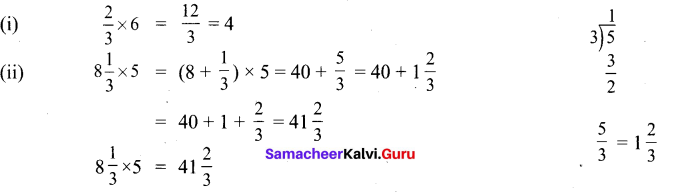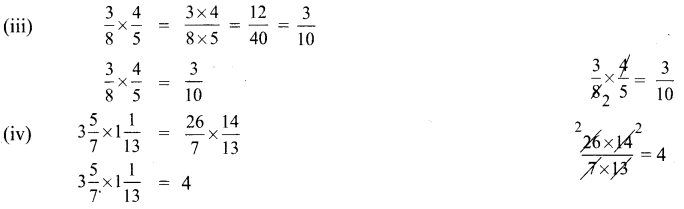Samacheer Kalvi 6th Maths Guide Term 3 Question 6.
Divide the following: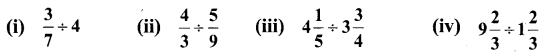Solution: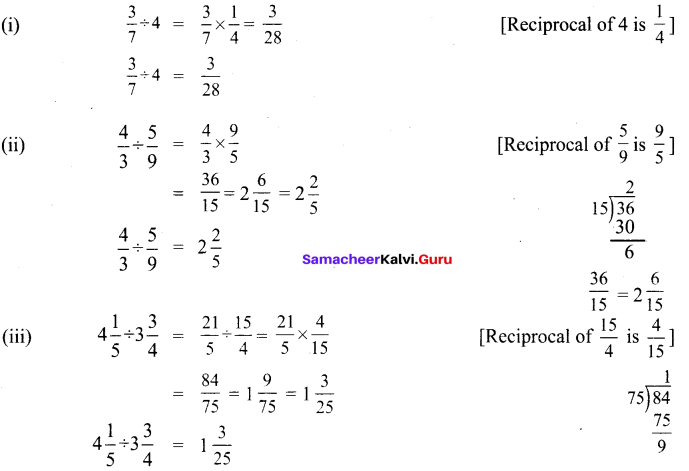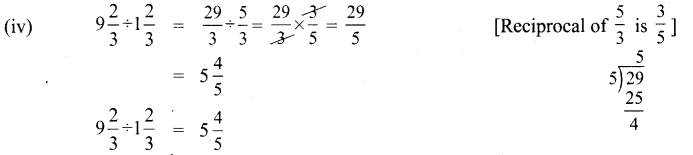6th Maths Term 3 Guide Question 7.
Gowri purchased 3$$\frac{1}{2}$$ kg of tomatoes, $$\frac{3}{4}$$ kg of brinjal and 1$$\frac{1}{4}$$ kg of onion, what is the total weight of the vegetables she bought?
Solution:
Weight of tomatoes Gowri purchased = 3$$\frac{1}{2}$$ kg
Weight of Brinjal purchased = $$\frac{3}{4}$$ kg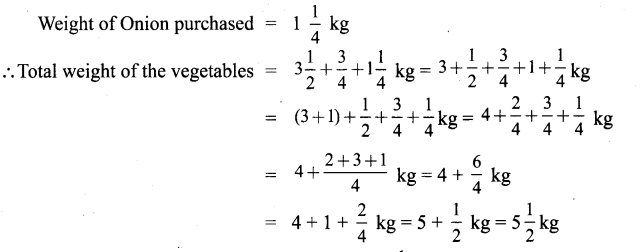Total weight of vegetables that Gowri purchased = 5$$\frac{1}{2}$$ kg

6th Term 3 Maths Guide Question 8.
An oil tin contains 3$$\frac{3}{4}$$ litres of oil of which 2$$\frac{1}{2}$$ litres of oil is used. How much oil is left over?
Solution: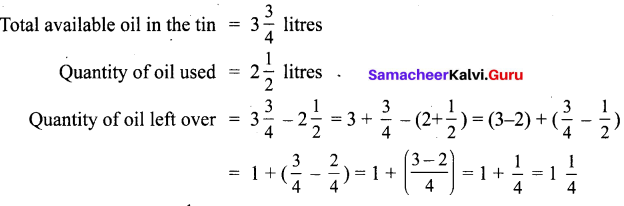Quantity of oil leftover = 1$$\frac{1}{4}$$ litres.

6th Maths Guide Term 3 Pdf Question 9.
Nilavan can walk 4$$\frac{1}{2}$$km in an hour. How much distance will he cover in 3$$\frac{1}{2}$$ hours?
Solution:
Distance walked by Nilavan in one hour = 4$$\frac{1}{2}$$ km.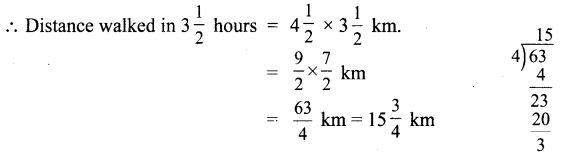Nilavan walks 15$$\frac{3}{4}$$ km in 3$$\frac{1}{2}$$ hours

6th Maths Term 3 Question 10.
Ravi bought a curtain of length 15$$\frac{3}{4}$$ m. If he cut the curtain into small pieces each of length 2$$\frac{1}{4}$$ m, then how many small curtains will he get?
Solution: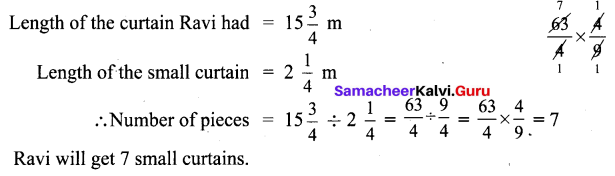6th Third Term Maths Guide Objective Type Questions

Samacheer Kalvi 6th Maths Book Term 3 Question 11.
Whcih of the following statement is incorrect?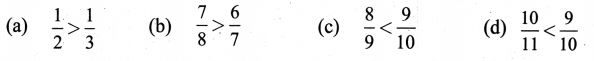Solution:
(d)$$\frac{10}{11}<\frac{9}{10}$$
Hint: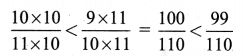Samacheer Kalvi 6th Maths Question 12.
The difference between $$\frac{3}{7}$$ and $$\frac{2}{7}$$ is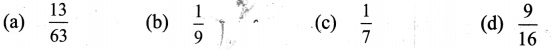Solution:
(a) $$\frac{13}{63}$$
Hint: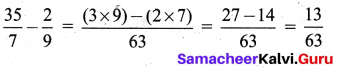6th Maths Term 3 Exercise 1.1 Question 13.
The reciprocal of $$\frac{53}{17}$$ is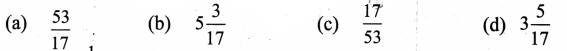Solution:
(c) $$\frac{17}{53}$$
Hint:
$$\frac{\frac{1}{53}}{\frac{53}{17}}=\frac{17}{53}$$

6th Standard Maths Guide Term 3 Question 14.
If $$\frac{6}{7}$$ = $$\frac{A}{49}$$, then the value of A is
(a) 42
(b) 36
(c) 25
(d) 48
Solution:
(a) 42

Samacheer Kalvi Guru 6th Maths Question 15.
Pugazh has been given four choices for his pocket money by his father. Which of the choices should he take in order to get the maximum money?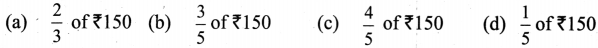Solution:
(c) $$\frac{4}{5}$$ of ₹150